# CBSE Physics Notes For Class 12 Current Electricity (Electric Current)

Posted on February 23rd, 2018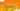## CBSE Physics Notes For Class 12 Current Electricity (Electric Current)

Physics notes class 12: The flow of electric charge through an electric called an electric current.  Electric current is basically caused by the flow of electrons or ions. It is denoted by I and its SI unit is ampere. Basically, we can say an electric Current is flow of charge in coulomb per second through any closed circuit. Thus, we have
I=Q/T, where Q is the amount of charge flowing through a circuit in time period T. Ammeter is the device used to measure electric current. Electric current is a scalar quantity though it has magnitude as well as the direction it does not follow the vector law of addition that’s why it is considered as the scalar.

CONVENTIONS: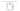As we know current flow from the positive terminal to negative terminal by convention but in reality, there are electrons flowing from negative terminals to positive terminals which causes a current. That whole convention was shown in the fig. given below: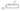OHMS LAW:

According to OHM’s Law, the electric current flowing through any conductor between any two points is directly proportional to the potential difference across those two points, that proportionality constant is resistance and the given equation is

I=V/R

Where ‘I’ is the current flowing through that conductor, V is the potential difference measured across the conductor, R is the resistance of that conductor and their SI units are amperes, Volts and Ohms respectively. The basic point of ohms law is that resistance R is the relation between the current flowing through the circuit and the potential difference across the terminals.

Watch animated videos on the same for better understanding and learning, click on NCERT Physics Class 12.

CURRENT DENSITY:

Current density is the measure of the density of an electric current. It is defined as the flow of electric current per cross-sectional area. It is a vector quantity. Its SI unit is amperes per square meter.

I = ∫ Ĵ.dÂ

Where ‘I’ is current in the conductor, Ĵ is the current density and dÂ is the differential cross-sectional area vector. The current density is directly proportional to the to the electric field in that particular medium and relation between the electric field and current density is obtained with the help of conductivity(σ) of the material. Hence, we can say,

Ĵ=σÊ

The reciprocal of conductivity is called as resistivity which is denoted by ρ and the above equation can be written as

Ĵ=Ê/ρ

Ê=Ĵρ

Learn with animated videos and make a boring topic interesting, for demos click Physics notes for class 12.

MEASUREMENT OF ELECTRIC CURRENT:

There are various methods which are used for the measurements of electric currents some of them are as follows:

1. Direct methods (current sense resistor method):

This method is called as a resistive method of current measurement. It is one of the most simple and linear method used for the measurement of current. In this method, the current sense resistor is placed in series with the current to be measured and the resultant current flows and the amount of power converted into the heat. This power produced provides the voltage signal and hence current can easily be measured.

1. Indirect methods (magnetic methods):

Indirect methods 0f measurement of current are also called as the magnetic method of measurement of electric current. They are done with the help of current transformers, Rogowski coil method and hall effect device. They are not as much simple and linear as compared to direct methods of measurement of current.

For NCERT Solutions for Class 12 Physics – Electric current, for details click CBSE 12 Science section.

MAGNETIC EFFECT OF ELECTRIC CURRENT:

Electric current produces a magnetic field. This phenomenon is called as electromagnetism i.e. whenever an electromagnetic coil having a large number circular turns of insulated turns behaves like a magnet when an electric current flows through it. And whenever the current stops, the coil will lose its magnetism. The direction of the magnetic field can be obtained by Maxwell Right and grip rule, the direction of magnetic field is given by the direction of fingers when thumb follows the direction of flow of current.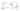Similarly, magnetism can also produce electric current. Whenever changing magnetic field applied across a conductor, the current will have induced inside a conductor basically an electromotive force is induced and in a suitable closed path, it causes an electric current.

ALTERNATING AND DIRECT CURRENT:

There are two types of electric current, they are alternating and direct current. When the electric charge moves periodically reverse direction called an alternating current. It is the form of electric current commonly delivered to our houses. The usual form of alternating current is a sine wave. Alternating current has various other applications as well.

When the flow of current is unidirectional or it moves in a system in one direction the current is called as direct current. The basic source of direct electric current is the battery. The direct was also called as galvanic current.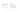.

Learning has become fun for students with Takshila Learning! As Takshila Learning offer HD quality animated videos. Moreover, we offer various quizzes, activity sheets, virtual practical’s, study material, and much more for 12th Physics. So, students develop their interest and understand topics very well. We offer animated courses with live classes as per the CBSE syllabus. Our main objective is to provide quality and result oriented education as well as guidance to the students’ perusing various courses, in order to achieve their goals.

For more inquires about Physics notes class 12, regular articles of 12th Physics on various topics , 12 Science, NCERT solutions class 12th, CBSE guide, sample papers, latest updates from CBSE Board, Enroll with Takshila Learning

Follow us on a Social media